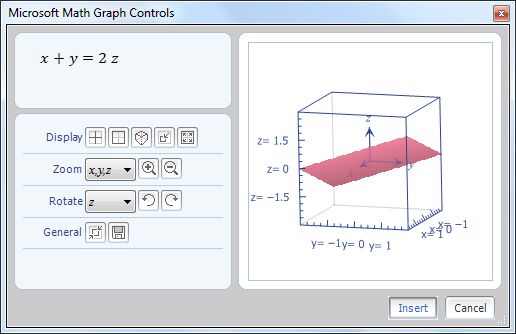How to Install Microsoft Mathematics add-in for Word and OneNote 2010

Though Microsoft Office 2010 especially its Word software does come with a better way of inserting equation and other complicated mathematical symbols, sometimes you still face problems inserting complex equation as well as plotting graphs. So you need to install the Microsoft Mathematics add-in to ease your work.

What can you do with Mathematics Add-in

• Compute standard mathematical functions such as roots and logarithms.
• Compute trigonometric functions such as sine and cosine.
• Find derivatives and integrals, limits, and sums and products of series.
• Perform matrix operations such as inverses, addition and multiplication.
• Perform operations on complex numbers.
• Plot 2-D graphs in Cartesian and polar coordinates.
• Plot 3-D graphs in Cartesian, cylindrical, and spherical coordinates.
• Solve equations and inequalities.
• Calculate statistical functions, such as mode and variance, on lists of numbers.
• Factor polynomials or integers.
• Simplify or expand algebraic expressions.1.Christine says:
2.Elisha Batuncang says: